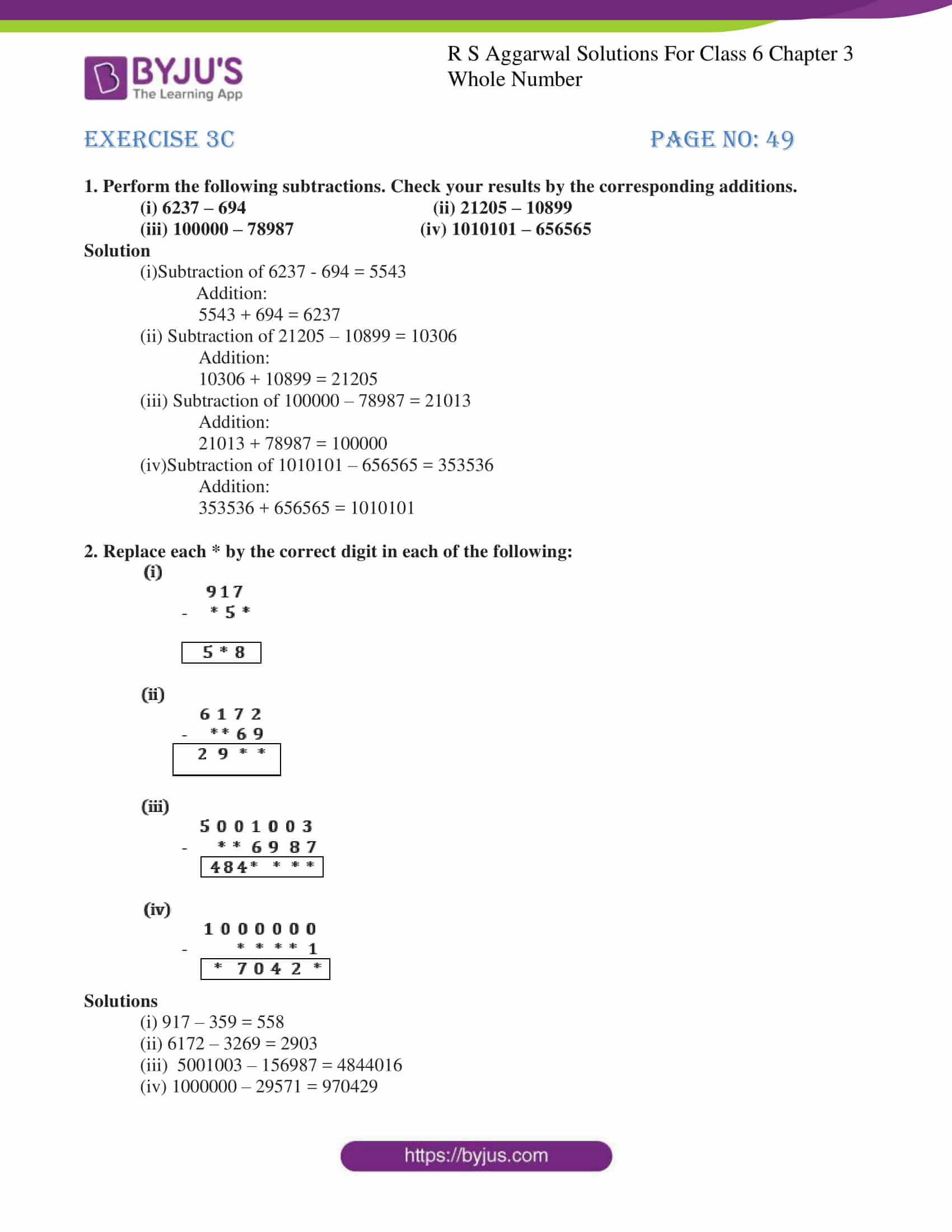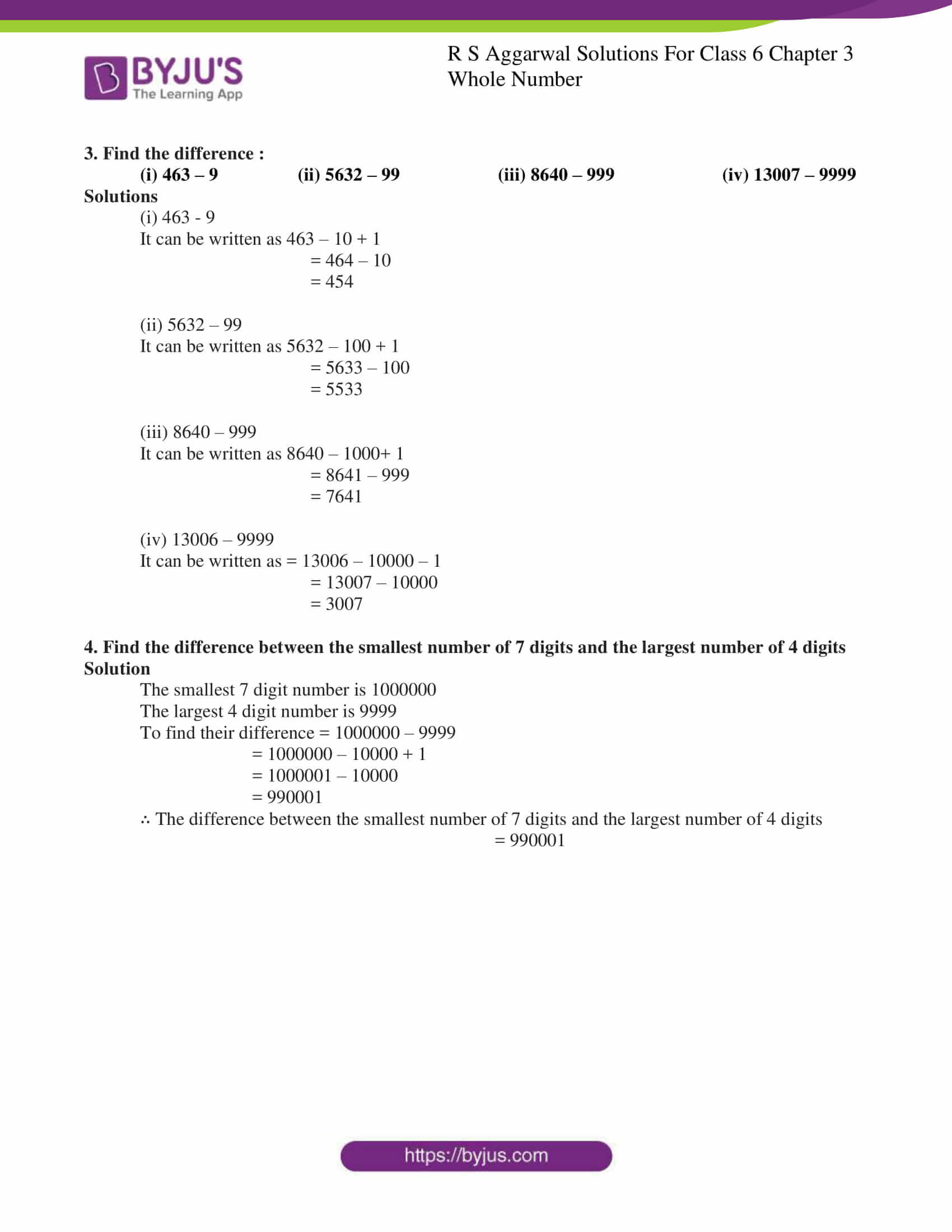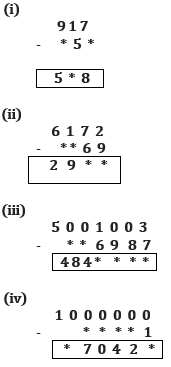# RS Aggarwal Solutions for Class 6 Chapter 3 Whole Numbers Exercise 3C

RS Aggarwal Solutions for Class 6, Chapter 3, Exercise 3C PDF are provided here. This exercise consists of topics like Subtraction in Whole Numbers and properties of subtraction. Students can refer to these solutions to get a brief idea about the topics more clearly. All questions of RS Aggarwal textbook are solved by expert Mathematicians, which is given in the PDF format. By using RS Aggarwal Solutions, students can easily achieve good marks in the examinations.

## Download PDF of RS Aggarwal Solutions for Class 6 Chapter 3 Whole Numbers Exercise 3C### Access answers to Maths RS Aggarwal Solutions for Class 6 Chapter 3 Whole Numbers Exercise 3C

1. Perform the following subtractions. Check your results by the corresponding additions.

(i) 6237 – 694 (ii) 21205 – 10899

(iii) 100000 – 78987 (iv) 1010101 – 656565

Solution

(i)Subtraction of 6237 – 694 = 5543

5543 + 694 = 6237

(ii) Subtraction of 21205 – 10899 = 10306

10306 + 10899 = 21205

(iii) Subtraction of 100000 – 78987 = 21013

21013 + 78987 = 100000

(iv)Subtraction of 1010101 – 656565 = 353536

353536 + 656565 = 1010101

2. Replace each * by the correct digit in each of the following:Solutions

(i) 917 – 359 = 558

(ii) 6172 – 3269 = 2903

(iii) 5001003 – 156987 = 4844016

(iv) 1000000 – 29571 = 970429

3. Find the difference :

(i) 463 – 9 (ii) 5632 – 99 (iii) 8640 – 999 (iv) 13007 – 9999

Solutions

(i) 463 – 9

It can be written as 463 – 10 + 1

= 464 – 10

= 454

(ii) 5632 – 99

It can be written as 5632 – 100 + 1

= 5633 – 100

= 5533

(iii) 8640 – 999

It can be written as 8640 – 1000+ 1

= 8641 – 999

= 7641

(iv) 13006 – 9999

It can be written as = 13006 – 10000 – 1

= 13007 – 10000

= 3007

4. Find the difference between the smallest number of 7 digits and the largest number of 4 digits

Solution

The smallest 7 digit number is 1000000

The largest 4 digit number is 9999

To find their difference = 1000000 – 9999

= 1000000 – 10000 + 1

= 1000001 – 10000

= 990001

∴ The difference between the smallest number of 7 digits and the largest number of 4 digits

= 990001